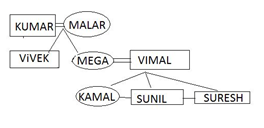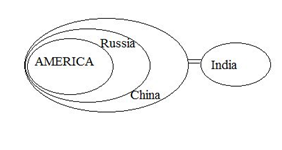# RRB ALP 2018 Practice Test Papers | Reasoning Questions (Day-48)

Dear Aspirants, Here we have given the Important RRB ALP & Technicians Exam 2018 Practice Test Papers. Candidates those who are preparing for RRB ALP 2018 can practice these Reasoning Questions to get more confidence to Crack RRB 2018 Examination.

[WpProQuiz 2617]

Click “Start Quiz” to attend these Questions and view Solutions

1). H is taller than I. I is shorter than J. J is taller than K and K is shorter than L. Who is the tallest?

1. H
2. G
3. L
4. K

2). Find the odd words from the given alternative.

1. AF: CH
2. BG: DI
3. CE: EG
4. DH: FK

3). Arrange these letters of each group to make a meaningful word and then find the odd one out.

1. PAPHY
2. OLEV
3. LOFERW
4. LUAHG

4). From the given alternatives, find the word which can be formed from the letters used in the given word.

PHENOMENON

1. PEN
2. LEMON
3. PHONE
4. OPEN

5). If S = 19, SUN = 44, then what is SUMMER equal to?

1. 80
2. 89
3. 99
4. 90

6). Which of the following Venn diagrams correctly illustrates the relationship among the classes Food items, Meals, Chocolate?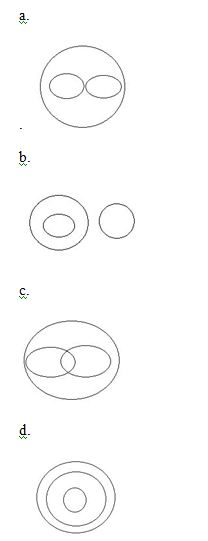7). Find the missing number

 2 4 6 12 6 8 10 24 12 16 20 ?
1. 50
2. 60
3. 48
4. 40

8). Find the missing number?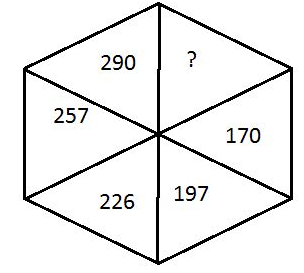1. 169
2. 144
3. 171
4. 325

9). Vimal is married to Mega. Sunil is brother of kamala. Kamala is the only daughter of Mega. Kumar is father of Mega, who has 3 children. Malar is married women, who is maternal grandmother of Sunil. Vivek is son of malar. Suresh is grandson of kumar. How suresh is related to Vivek?

1. Brother
2. Brother in law
3. Cousin
4. Nephew
1. Read the Statement and Find which of the following conclusion definitely not follow the statement

Statement:

1. All America is Russia
2. All Russia is china
3. Some china is not in India
1. Some America is china
2. Some Russia is America
3. All India is America is possibility
4. Some India is China

H>I>J>K>L

A+4F: C+4H

B+4G: D+4I

C+1E: E+1G

D+4H: F+4K

 Given words Meaningful words PAPHY HAPPY OLEV LOVE LOFERW FLOWER LUAHG LAUGH

Except Flower all others are Feelings. So, Flower is the odd one out.

Lemon cannot be formed from the word PHENOMENON. Because L is not present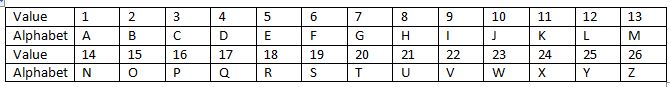In Alphabetical Order

S is in 19th place. So S = 19

In the same way

S=19; U= 21; N=14

= 19+ 21+ 14 = 44

S=19; U=21; M=13; M=13; E=05; R=18

= 19+21+13+13+05+18 = 89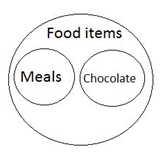2 + 4 + 6 = 12

6 + 8 + 10 = 24

12 + 16 + 20 = 48

(132+1) = 169 + 1 = 170

(142+ 1) = 196 + 1 = 197

(152 + 1) = 225 + 1 = 226

(162 + 1) = 256 + 1 = 257

(172 + 1) = 289 + 1 = 290

(182 + 1) = 324 + 1 = 325